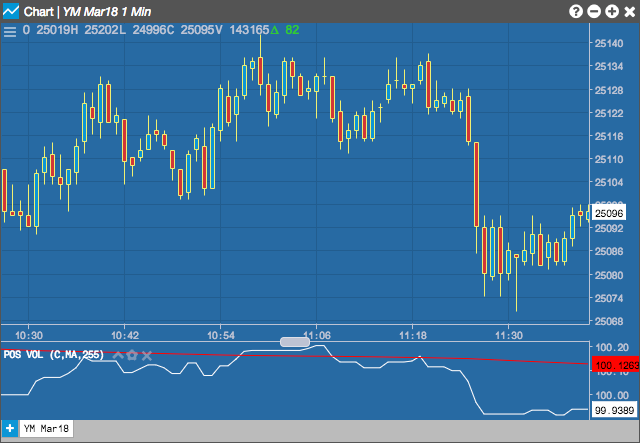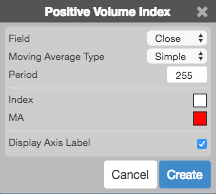Charts

# Positive Volume Index (PVI)

The Positive Volume Index (PVI), as well as the Negative Volume Index (VMI), indicator tracks the changes in the number of transactions, or trading volume, of an instrument. Price changes on decreasing trading volume are considered to be a positive indicator, while price changes during periods of increased trading volume are considered to be a negative indicator. The idea behid the indicator is that well-informed traders ("smart money") are involved when trading volumes decrease, while increasing trading volumes can indicate a follow-the-crowd mentality ("not-so-smart money").

The PVI displays what the "not-so-smart money" is doing; while the NVI tracks what the "smart money" is doing.## Configuration Options• Field: Price or combination of prices to use as the base for average calculations. Possible values include:
• Open
• High
• Low
• Close
• HL/2 $$\left ( \frac{High + Low}{2} \right )$$
• HLC/3 $$\left ( \frac{High + Low + Close}{3} \right )$$
• HLCC/4 $$\left ( \frac{High + Low + Close + Close}{4} \right )$$
• OHLC/4 $$\left ( \frac{Open + High + Low + Close}{4} \right )$$
• Moving Average Type: Type of moving average to use in the calculations:
• Simple
• Exponential
• Time Series
• Triangular
• Variable
• VIDYA
• Weighted
• Welles Winder
• Hull
• Double Exponential
• Triple Exponential
• Period: Number of bars to use in the calculations.
• Color Selectors: Colors to use for graph elements.
• Display Axis Label: Whether to display the most recent value on the Y axis.

## Formula

Positive volume index is calculated as follows:

• If today's volume is greater than yesterday's volume: $PVI = PVI_\text{yesterday} + \left( \frac {FieldPrice - FieldPrice_\text{yesterday}}{FieldPrice_\text{yesterday}} \times PVI_\text{yesterday} \right )$
• Otherwise: $PVI = PVI_\text{yesterday}$